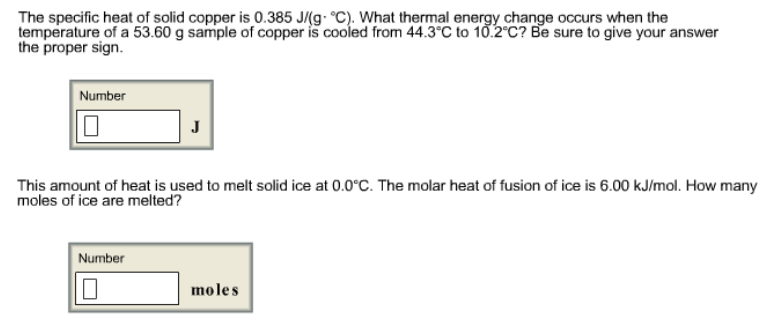# Problem: The specific heat of solid copper is 0.385 J/(g • °C). What thermal energy change occurs when the temperature of a 53.60 g sample of copper is cooled from 44.3 °C to 10.2 °C? Be sure to give your answer the proper sign. This amount of heat is used to melt solid ice at 0.0 °C. The molar heat of fusion of ice is 6.00 kJ/mol. How many moles of ice are melted?

###### FREE Expert Solution
92% (263 ratings)###### Problem Details

The specific heat of solid copper is 0.385 J/(g • °C). What thermal energy change occurs when the temperature of a 53.60 g sample of copper is cooled from 44.3 °C to 10.2 °C? Be sure to give your answer the proper sign.

This amount of heat is used to melt solid ice at 0.0 °C. The molar heat of fusion of ice is 6.00 kJ/mol. How many moles of ice are melted?